# 推荐系列（六）：深层神经网络模型（1）uncle_ll 2019-07-31

• 使用侧面特征困难（即查询ID /项目ID以外的任何特征）。因此，只能使用训练集中存在的用户或项目来查询模型。
• 建议的相关性。每个人都倾向于推荐受欢迎的项目，特别是在使用点积作为相似性度量时。最好是能够捕获特定的用户兴趣。

## Softmax DNN推荐

• 输入是用户查询。

### 输入

DNN的输入可包括：

• 密集特征（例如，观看自上次观看以来的时间和时间）
• 稀疏特征（例如，观看历史记录和国家/地区）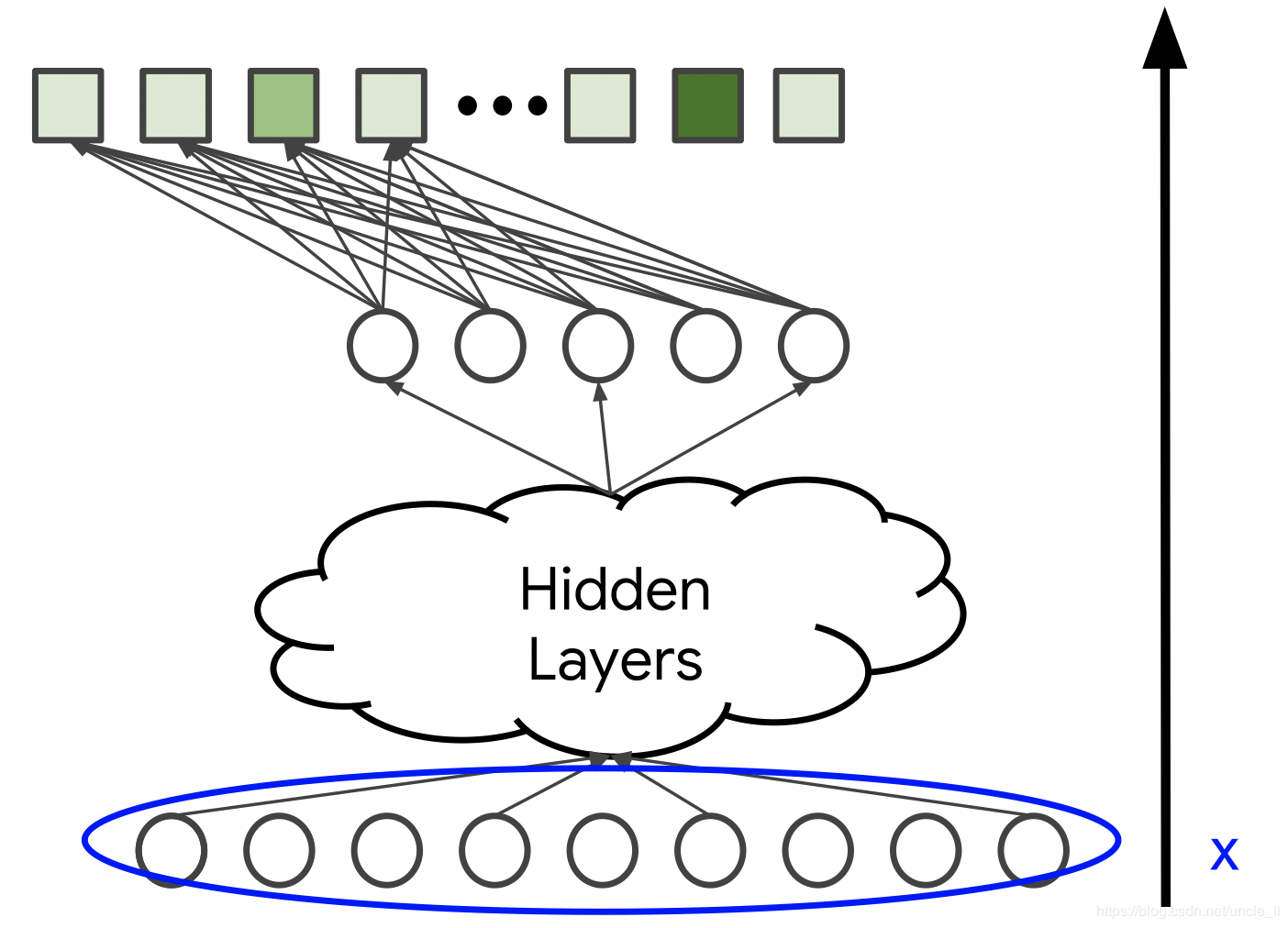### 模型架构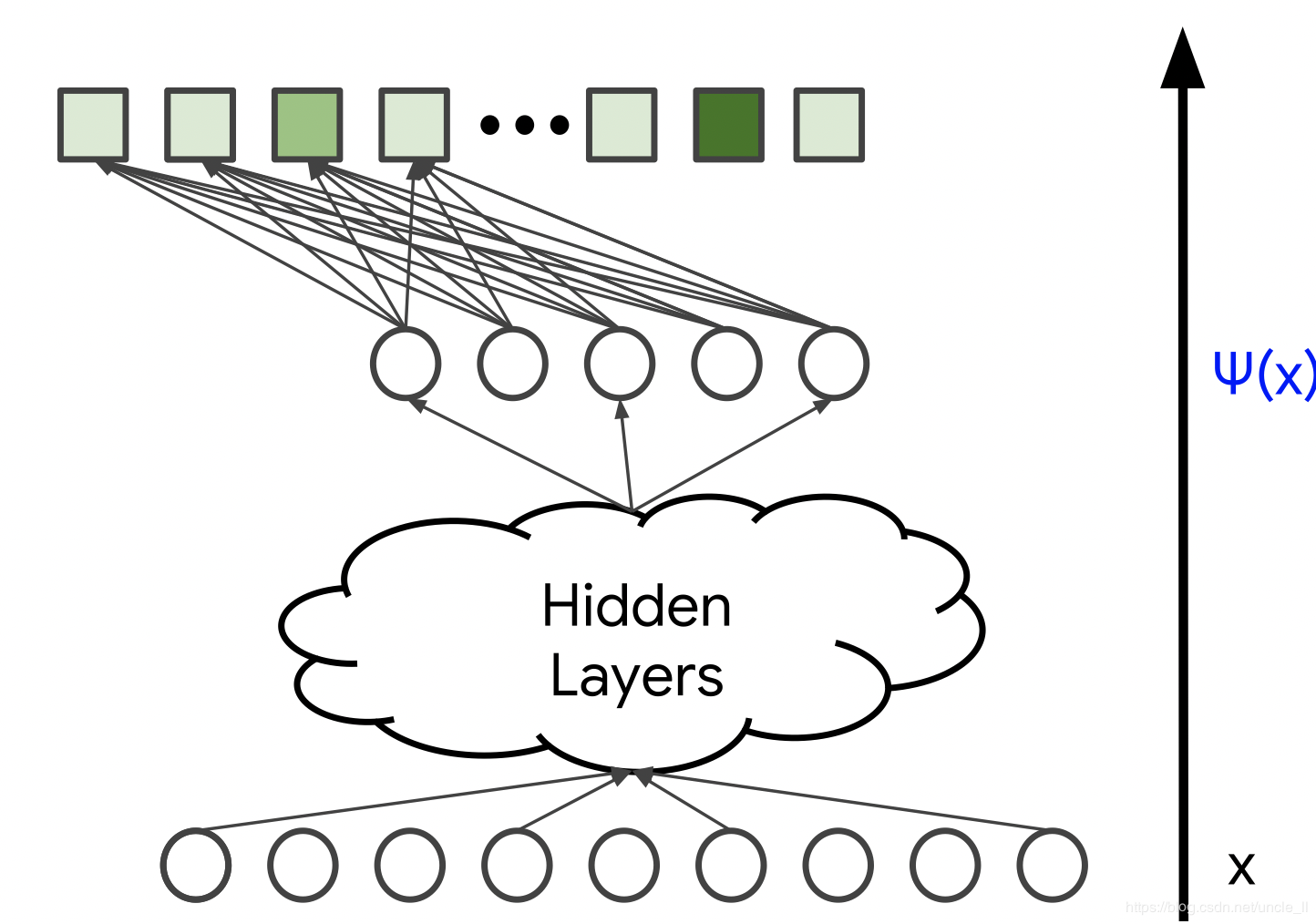### Softmax输出：预测的概率分布

• $h : \mathbb R^n \to \mathbb R^n$ 是softmax函数，由下式给出 $h(y)_i=\frac{e^{y_i}}{\sum_j e^{y_j}}$
• $V \in \mathbb R^{n \times d}$是softmax层的权重矩阵。

softmax层映射得分矢量$y \in \mathbb R^n$有时称为 (logits）为概率分布。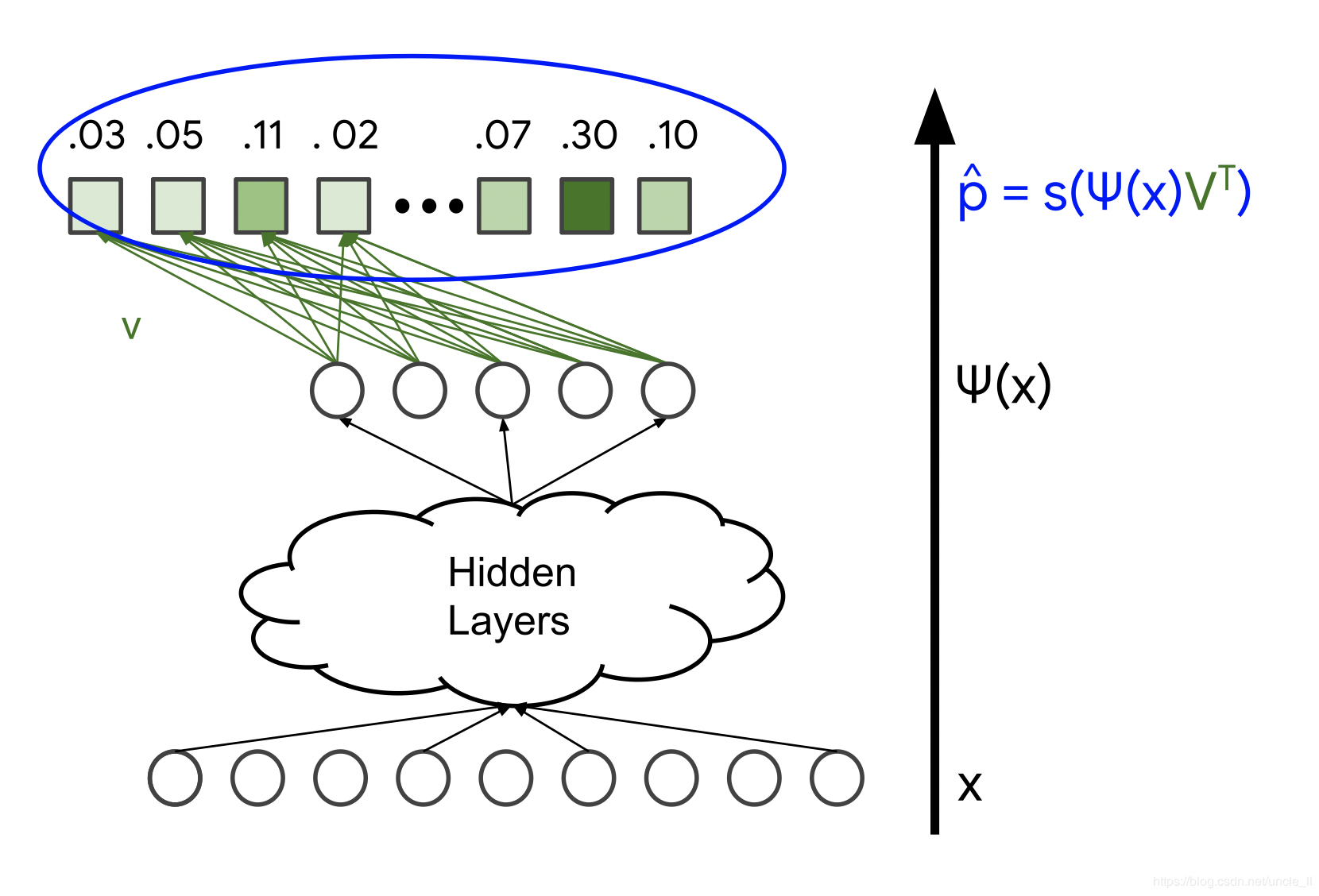softmax这个名字是一个单词的游戏。“硬”（hard）表示将最大值的概率1分配给得分最高的项目 ÿ。相比之下，softmax为所有的项目分配非零概率，但是具有更高分数的项目其概率也越高。当 α→∞，分数缩放时，softmax $h(\alpha y)$ 收敛到"硬"最大值。

### 损失函数

• $\hat p$，softmax层的输出（概率分布）；
• $p$，真值，真实标签，可以表示为归一化的多热分布（概率矢量）；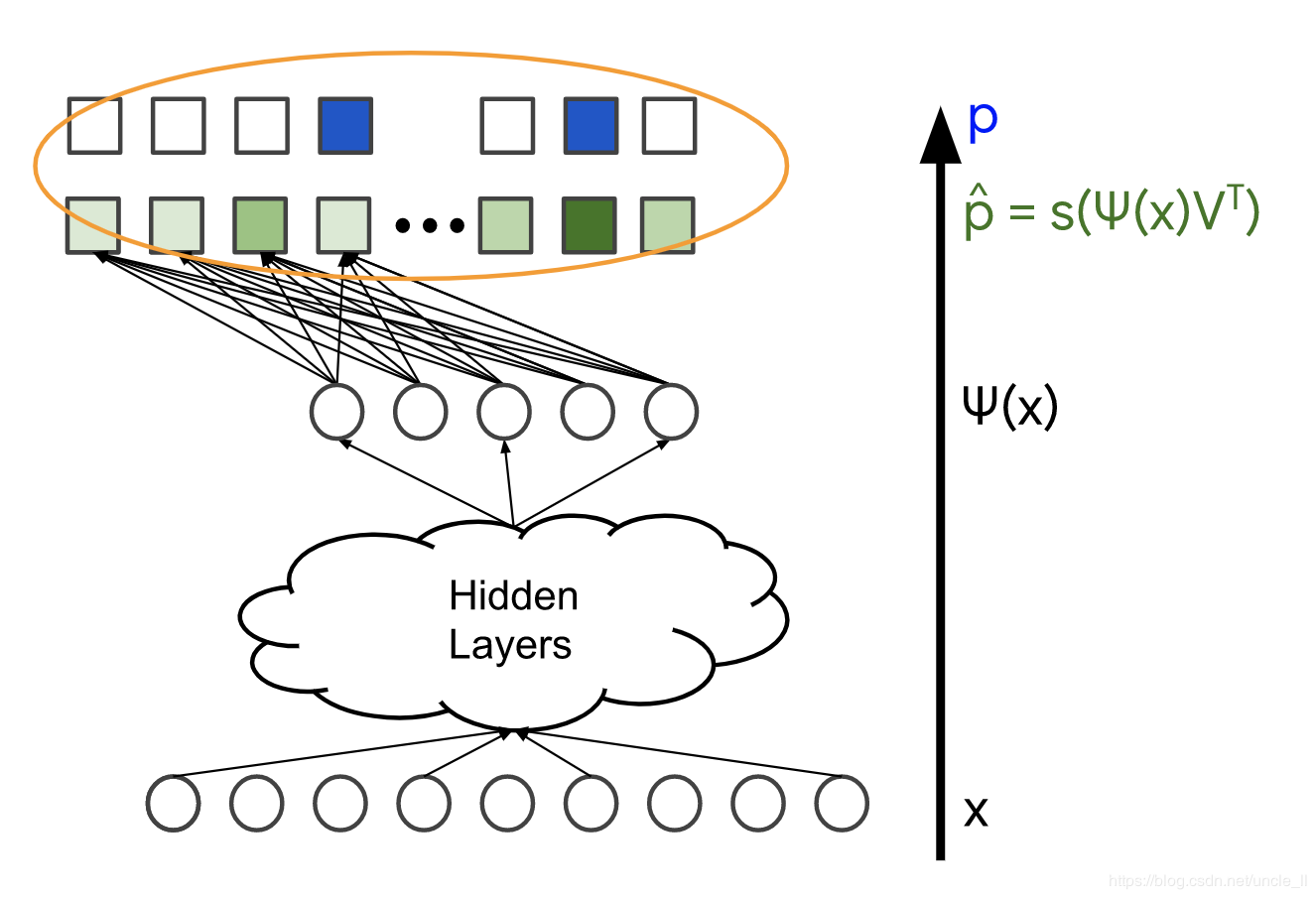### Softmax嵌入

• $\psi(x) \in \mathbb R^D$是最后一个隐藏层的输出，称之为查询$x$嵌入；
• $V_j \in \mathbb R^d$是将最后一个隐藏层连接到输出j的权重向量，称之为项目$j$嵌入；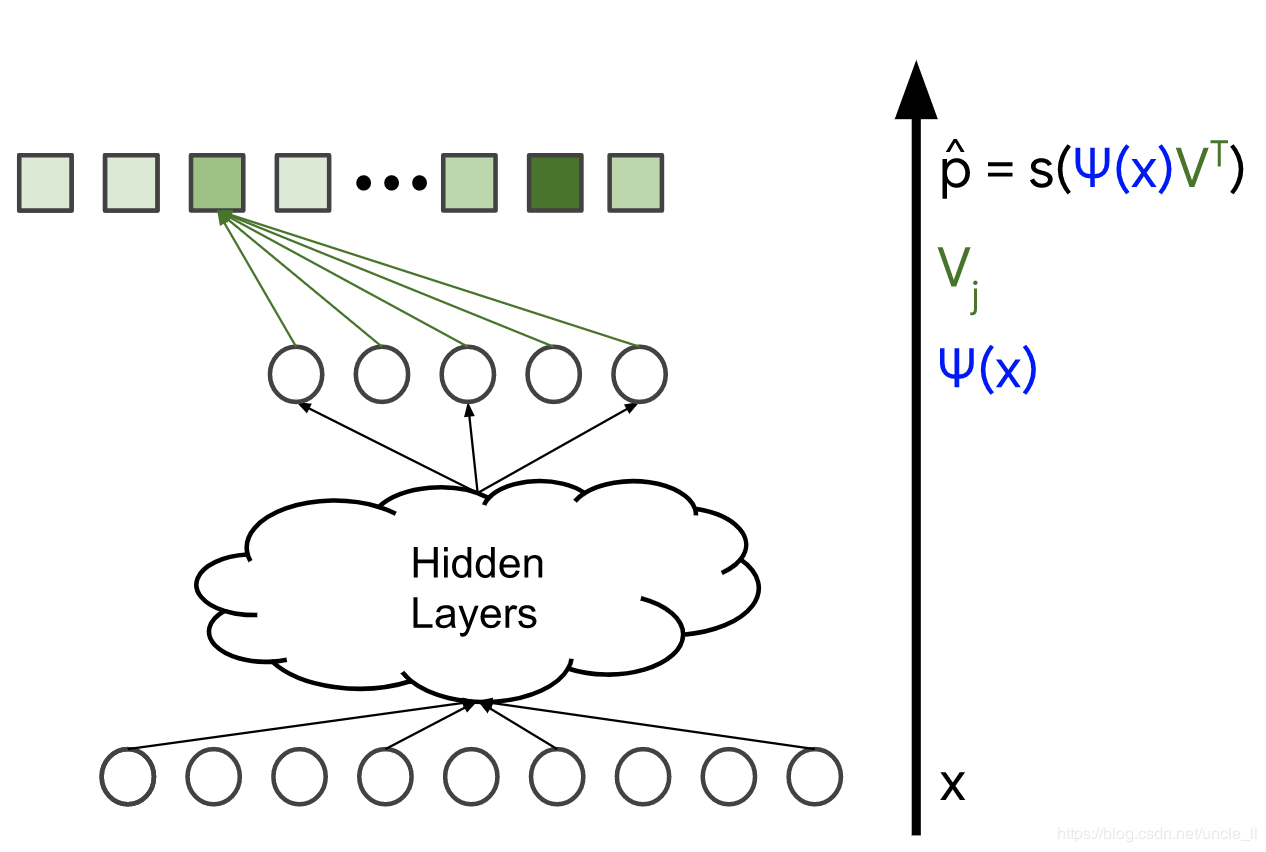## DNN和矩阵分解

### 使用物品特征

• 一个神经网络映射查询特征$x_{query}$到查询嵌入$\psi(x_{\text{query}}) \in \mathbb R^d$
• 一个神经网络映射项目特征$x_{item}$到项目嵌入$\phi(x_{\text{item}}) \in \mathbb R^d$

617人浏览
2020-07-13

38199人浏览
2017-10-04 23:31:42

13858人浏览
2018-02-18 22:00:00

2578人浏览
2017-11-17 23:09:00

1702人浏览
2017-05-02 20:55:00

4234人浏览
2018-03-07 10:18:15
0
0
987